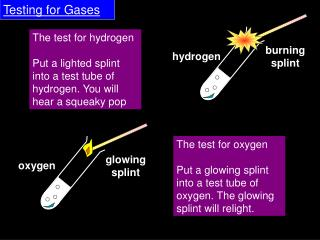DownloadDownload PresentationTesting for Gases

# Testing for Gases

Télécharger la présentation## Testing for Gases

- - - - - - - - - - - - - - - - - - - - - - - - - - - E N D - - - - - - - - - - - - - - - - - - - - - - - - - - -
##### Presentation Transcript

1. Testing for Gases burning splint hydrogen The test for hydrogen Put a lighted splint into a test tube of hydrogen. You will hear a squeaky pop The test for oxygen Put a glowing splint into a test tube of oxygen. The glowing splint will relight. glowing splint oxygen

2. Testing for Gases burning splint hydrogen The squeaky pop test gives out energy so how do we describe it? We need to be able to draw an energy level diagram to show this reaction.

3. Testing for Gases burning splint hydrogen The test for hydrogen involves reacting hydrogen with oxygen. Write the word and balanced symbol equation for this reaction.

4. How does a hydrogen fuel cell work?

5. Calculating the energy Bond energies can be used to calculate the amount of energy given out by the reaction in a hydrogen fuel cell. Energy is taken in to break bonds, and released when bonds are created. Bond Bond Energy (kJ) H–H 432 O=O 498 O–H 463 energy for bond-breaking energy from bond-making = 2H–H + O=O = 4H–O = 2 × 432 + 498 = 4 × 463 = 1362kJ = 1852kJ total energy change = energy in – energy out = 1362kJ – 1852kJ = –490kJ

6. Energy level diagram

7. Where does the hydrogen come from? At the moment the hydrogen for fuel cells is extracted from crude oil. It may be possible to use solar power to extract hydrogen from water, but this method is still under development. What are the advantages and disadvantages of each method of making hydrogen?

8. Advantages of fuel cells The big advantage of hydrogen fuel cells is that the only emission they produce is water vapour. What are the other advantages? • Hydrogen has a low density, making vehicles light and efficient. • Hydrogen gives out more energy per gram than conventional fuels. What are some of the disadvantages of fuel cell technology? • Production of hydrogen from fossil fuels produces carbon dioxide – a greenhouse gas. • Hydrogen is highly flammable, making it risky to store. • Hydrogen is not cheap!

9. Fuel cells activity